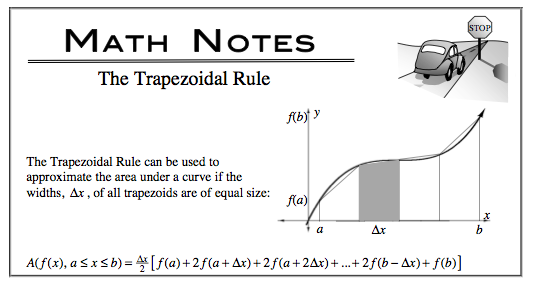### Home > CALC > Chapter 5 > Lesson 5.4.1 > Problem5-147

5-147.

The function $f$ is continuous on the closed interval $[2, 8]$ and has values that are in the table to the right.

1. Using the sub-intervals $[2, 5]$, $[5, 7]$ and $[7, 8]$, what is the trapezoidal approximation of $\int _ { 2 } ^ { 8 } f ( x ) d x$?

2. On what sub-interval is the average slope a minimum?

1. $[2, 5]$

1. $[5, 7]$

1. $[7, 8]$Test all answer choices and choose the lowest value. Recall that average slope is akin to saying average rate of change (AROC).